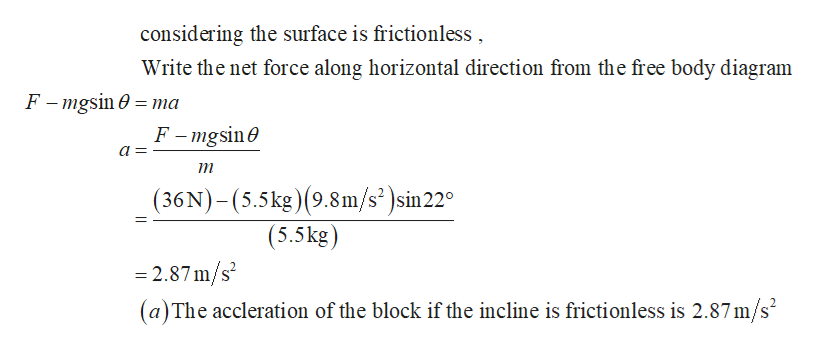Question
5 views

A block of mass

m = 5.5 kg

is pulled up a

θ = 22°

incline as in the figure below with a force of magnitude F = 36 N.

(a) Find the acceleration of the block if the incline is frictionless. (Give the magnitude of the acceleration.)
m/s2

(b) Find the acceleration of the block if the coefficient of kinetic friction between the block and incline is 0.12. (Give the magnitude of the acceleration.)
m/s2
check_circle

Step 1

The below diagram represents the free body diagram of the block .

Step 2help_outlineImage Transcriptioncloseconsidering the surface is frictionless Write the net force along horizontal direction from the free body diagram F-mgsin 0 ma F -mgsin т (36N)-(5.5kg)(9.8m/s )sin 22° (5.5kg) =2.87 m/s (a)The accleration of the block if the incline is frictionless is 2.87m/s fullscreen

### Want to see the full answer?

See Solution

#### Want to see this answer and more?

Solutions are written by subject experts who are available 24/7. Questions are typically answered within 1 hour.*

See Solution
*Response times may vary by subject and question.
Tagged in

### Newtons Laws of Motion